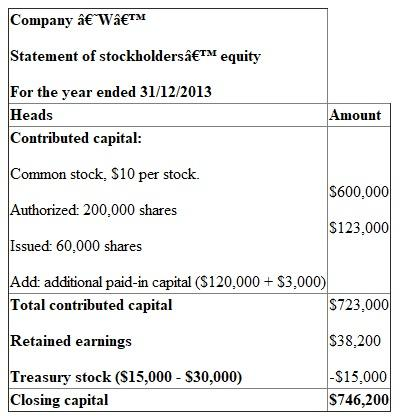# Quiz 11: Stockholders Equity

1. Assets: The properties owned by a business of having future benefits are assets. The assets of less than one year of benefit are current assets. The assets having more than one year of benefit are noncurrent assets. Liabilities: The debts of a business are known as liabilities. The liabilities which are going to meet within a year are current liabilities. The liabilities which will remain in the business beyond the current year are noncurrent liabilities. Stockholders' equity: This is the ownership rights in the company. Shareholders invest in the company builds stockholders' equity. The earnings in way of profit is also creates stockholders' equity. Debit: Increase in assets and decrease in liabilities and stockholders' equity are debits. Credit: Decrease in assets and increase in liabilities and stockholders' equity are credits. a. Cash: Cash is an asset and increases by the issue of preferred stock. Preferred stock: The shares of dividend-preference are preferred stock. It increases stockholders' equity, therefore, credited. b. Treasury stock: The shares which are repurchased from market by the company are treasury stocks. It reduces stockholders' equity, therefore, debited. Cash: The cost of repurchasing reduces assets, therefore, credited in cash. c. Dividend declared: This is the amount of cash dividend declared to outstanding stockholders. It reduces stockholders' equity, therefore, debited. Dividend payable: The dividend amount not yet paid is dividend payable. It creates liability, therefore, credited. d. Dividend payable: At the time of payment this account should be debited to nullify the earlier obligation. Cash: Payment out of cash, reduces assets, therefore, credited. Prepare journal entries of transactions of company 'N' as below: Company 'N' Journal entries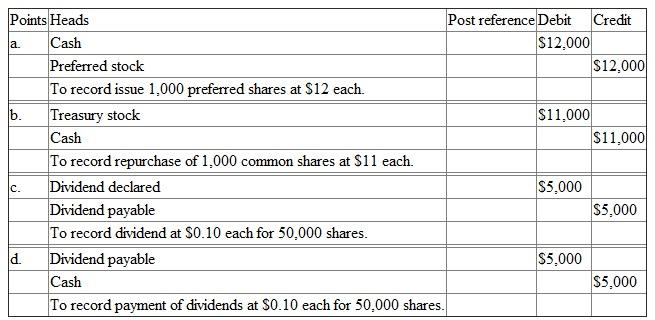Each transaction has equal amount of debit and credit. In each case multiply the number of shares with its price to get the respective amount. 2. A common shareholder must prefer the issuance of preferred stock to protect the voting rights. Common shareholders: The ordinary shareholders having voting rights, but not having dividend-distribution preference are common shareholders. Since preferred stockholders do not have the voting rights, a common shareholder of the company likes to have issue shares to them. 3. Prepare accounting equations of transactions of company 'N' as below: Company 'N' Accounting equations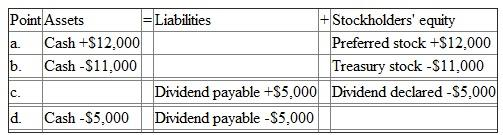a. Cash increases assets and the preferred stock increases stockholders' equity. Therefore, the equation is satisfied. b. Cash reduces assets and the treasury stock reduces stockholders' equity. Therefore, the equation is satisfied. c. The amount of dividend increases liabilities and decreases stockholders' equity. Therefore, the equation is satisfied. d. The payment of dividend decreases liabilities and decreases assets. Therefore, the equation is satisfied. 4. Return on equity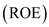: This is the net income per average stockholders' equity. Net income should be divided by average stockholders' equity to get ROE. Stockholders' equity is the amount of ownership rights of investors to the company. a. Decrease in ROE: increase in preferred stock of \$12,000 increases the average stockholders' equity. Since the denominator increases, it leads to decrease ROE b. Increase in ROE: increase in treasury stock of \$11,000 decreases the average stockholders' equity. Since the denominator decreases, it leads to increase ROE. c. Increase in ROE: increase in dividend declared of \$5,000 decreases the average stockholders' equity. Since the denominator decreases, it leads to increase ROE. d. Not affected in ROE: Payment of dividend has no connection with the stockholders' equity. Therefore, there will be no change in ROE.
1. Accounting equation: Establishing the relationship between assets, liabilities, and stockholders' equity of an accounting transaction is its accounting equation. The accounting equation is as below: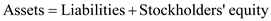The left side of the above equation must be equal to the right side. Assets: The properties owned by a business of having future benefits are assets. The assets of less than one year of benefit are current assets. The assets having more than one year of benefit are noncurrent assets. Liabilities: The debts of a business are known as liabilities. The liabilities which are going to meet within a year are current liabilities. The liabilities which will remain in the business beyond the current year are noncurrent liabilities. Stockholders' equity: This is the ownership rights in the company. Shareholders invest in the company builds stockholders' equity. The earnings in way of profit is also creates stockholders' equity. Explain each transaction appeared in accounting equation and journal as below: On 15/01: Cash of \$50,000 is received. Cash is an asset and increases, therefore, added as an asset and debited in journal. Common stock: The stock of ordinary shareholder is common stock. It is a part of stockholders' equity, therefore, added there and credited in journal. \$1 should be multiplied by 5,000 shares.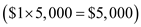. Additional paid-in capital: This is excess amount of issue. Subtract the common stock from cash to get the figure.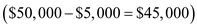. 15/02: Spent \$33,000 for share repurchase. It decreases cash, therefore, deducted from assets and credited in journal. Treasury stock: The repurchasing shares are treasury stocks. It is a part of stockholders' equity by a deduction, therefore decreases there and debited in journal. 15/03: Treasury stocks of 2,000 shares are reissued. It increases cash and assets, therefore added and debited in journal. Treasury stock: Since 3,000 shares were acquired of \$33,000. Per share price would be a division.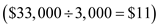. Now the value of 2,000 shares would be a multiplication of 2,000 shares and \$11.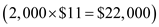. This amount reduces treasury stock. It leads to increase in stockholders' equity and the credit effect in journal. Additional paid-in capital: The difference of \$24,000 and \$22,000, which is \$2,000, is the additional amount receiving. It increases stockholders' equity and credited in journal. 15/08: Treasury stocks of 600 shares are reissued. It increases cash and assets, therefore added and debited in journal. Treasury stock: Since 3,000 shares were acquired of \$33,000. Per share price would be a division.. Now the value of 600 shares would be a multiplication of 600 shares and \$11.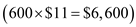. This amount reduces treasury stock. It leads to increase in stockholders' equity and the credit effect in journal. Additional paid-in capital: The difference of \$6,600 and \$4,600, which is \$2,000, is the balance amount not receiving. It decreases stockholders' equity and debited in journal. 15/09: Declared dividend: This is the amount of cash dividend declared to outstanding stockholders. Outstanding stockholders are common stockholders by a deduction of balance treasury stock. Since 2,600 shares were reissued from treasury stock of \$5,000, the balance would be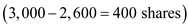. Outstanding shares of the company are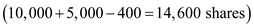. Since the price is \$1, it should be multiplied by 14,600 to get \$14,600 as the declared dividend amount. It reduces stockholders' equity, therefore decreases there and debited in journal. Dividend payable: This is a liability of the company for paying dividend. Dividend once declared it should be paid. It creates liability, therefore increases in liability section and credited in journal. 01/10: Received cash of \$100,000. It increases cash and debited in journal. Bond payable: This is the way of procuring money from market. It's a liability and should be increased. Bond payable should be credited in journal. Multiply \$101 and 100 bonds and get \$10,100. Premium on bond: This is the excess collection by issuing bond. Subtract \$10,100 from \$100,000 and get \$89,900. It increases stockholders' equity, therefore added there and credited in journal. 03/10: Accounts receivable: This is the outstanding amount of receivable. It reduces by the amount of write-off. Reduce assets by \$500 and make credit in journal. Allowance for doubtful debts: This is the provision of non-collection. It reduces in liability, therefore, debited in journal. 31/12: Depreciation : This is the yearly reduction of asset. Treat it as an expense. Stockholders' equity should be reduced and debited in journal. Accumulated depreciation : This is the cumulative amount of depreciation in each year. Charging depreciation increases accumulated depreciation. Therefore, increase it in liability and credit it in journal. Prepare accounting equations of transactions during 2013 as below: Company 'A' Accounting equations During the year 2013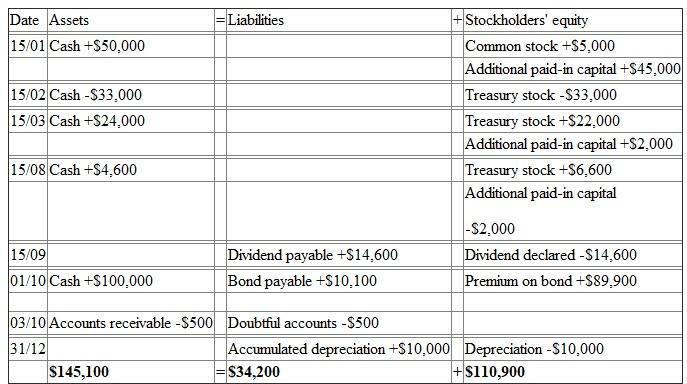Both sides of equation at the end are showing equal figure, \$145,100. 2. Prepare journal entries of transactions during 2013 as below: Company 'A' Journal entries During the year 2013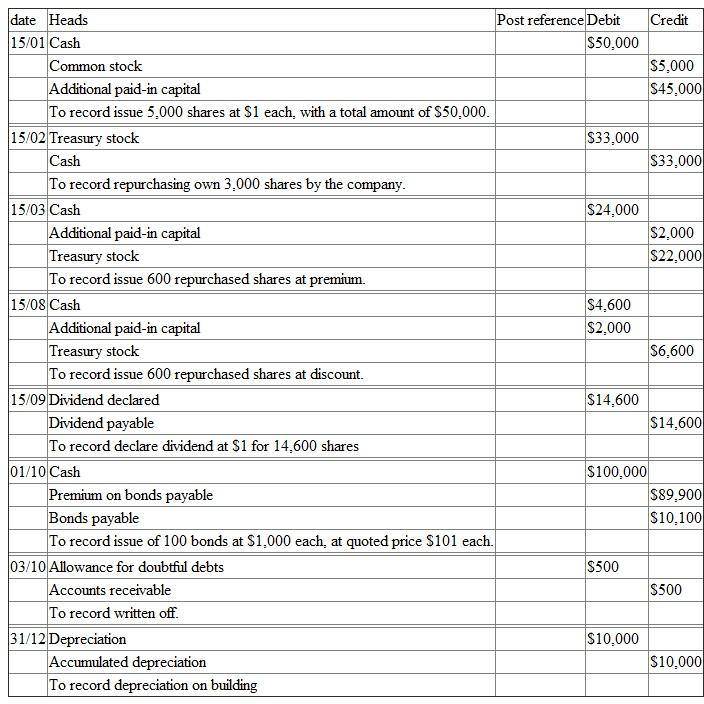Each transaction has equal amount of debit and credit. 3. Noncurrent liability: The liability of a company not going to meet within a year is noncurrent liability. Balance sheet: The statement of presenting assets, liabilities, and stockholders' equity at the end of a period is a balance sheet. Prepare a partial balance sheet as at 31/12/2013 below: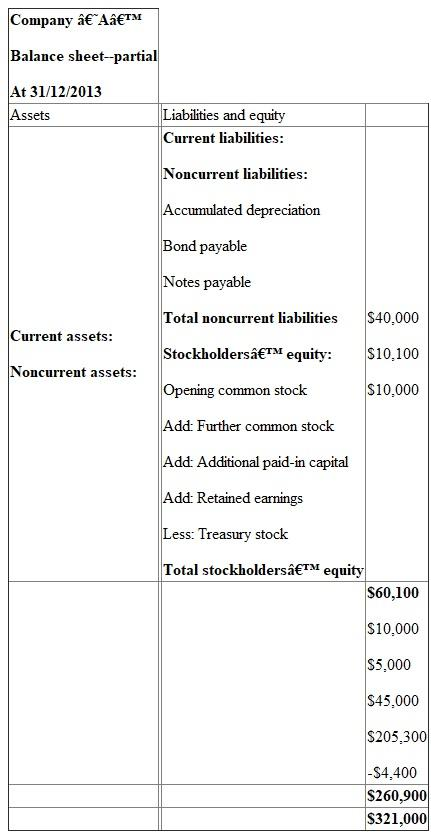The noncurrent liabilities are accumulated depreciation, bond payable, and notes payable. Stockholders' equity must be included with retained earnings, and treasury stock. Retained earnings: The accumulated earning till date is retained earnings. The opening earnings should be added with net income during the year, \$20,000. Then add premium on bond, \$89,900, and deduct dividend declared, \$14,600, and depreciation, \$10,000. The total amount would be \$205,300. Treasury stock: The repurchased stock of \$33,000 should be deducted by reissued of \$22,000 and \$6,600 to get \$4,400.
1. The effect of each transaction on total assets, liabilities, and stockholders' equity is shown below: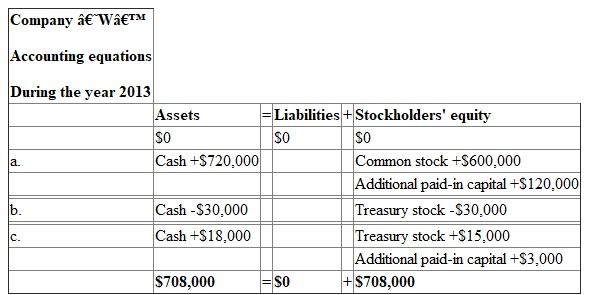This is the first year of operation. Therefore, the company has no opening balances and both the sides of equation at the end are showing equal figure of \$708,000. 2. The journal entries to record the transactions during 2013 as given below: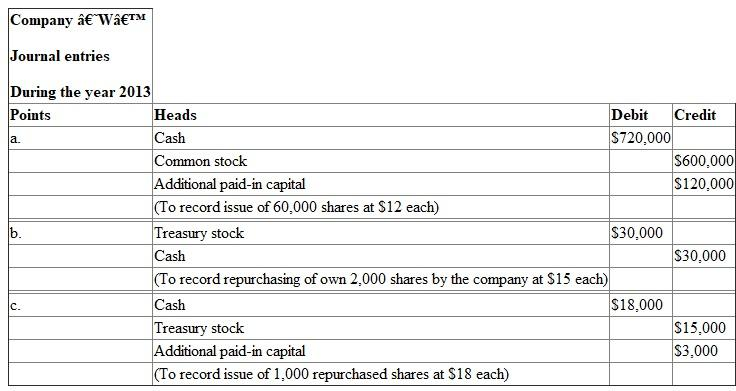3. The stockholders' equity section of company 'W' for the year ended 31 st December 2013 is given below: